# L-Norms as Loss Function

L

In linear algebra, functional analysis, and related areas of mathematics, a norm (l-norms) is a function that assigns a strictly positive length or size to each vector in a vector space—save for the zero vector, which is assigned a length of zero. A seminorm, on the other hand, is allowed to assign zero length to some non-zero vectors (in addition to the zero vector).

L1-Norm loss function is known as least absolute deviations (LAD).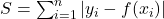It is basically minimizing the sum of the absolute differences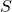between the target value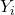and the estimated values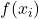.

L2-Norm loss function is known as least squares error (LSE).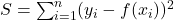It is basically minimizing the sum of the square of the differencesbetween the target valueand the estimated valuesDifferences between L1-L2 norm

The differences of L1-norm and L2-norm as a loss function are the following.

 L1-norm L2-norm Robust Not robust Unstable solution Stable solution Possible multiple solutions Only one solution